# inverse of a matrix

Inverse of a Matrix
Matrix Inverse
Multiplicative Inverse of a Matrix

For a square matrix A, the inverse is written A-1. When A is multiplied by A-1 the result is the identity matrix I. Non-square matrices do not have inverses.

Note: Not all square matrices have inverses. A square matrix which has an inverse is called invertible or nonsingular, and a square matrix without an inverse is called noninvertible or singular.

AA-1 = A-1A = I

 Example: For matrix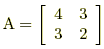, its inverse is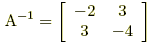since AA-1 =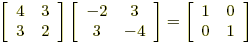and A-1A =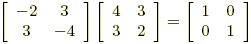.

Here are three ways to find the inverse of a matrix:

 1. Shortcut for 2x2 matricesFor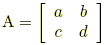, the inverse can be found using this formula: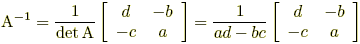Example: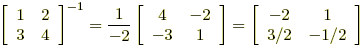2.Augmented matrix methodUse Gauss-Jordan elimination to transform [ A | I ] into [ I | A-1 ]. Example: The following steps result in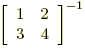.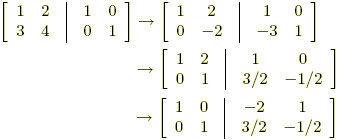so we see that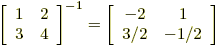. 3. Adjoint methodA-1 =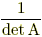(adjoint of A)   or   A-1 =(cofactor matrix of A)T Example: The following steps result in A-1 for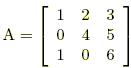.The cofactor matrix for A is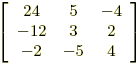, so the adjoint is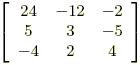. Since det A = 22, we get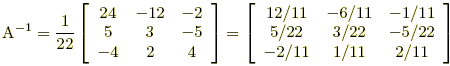.